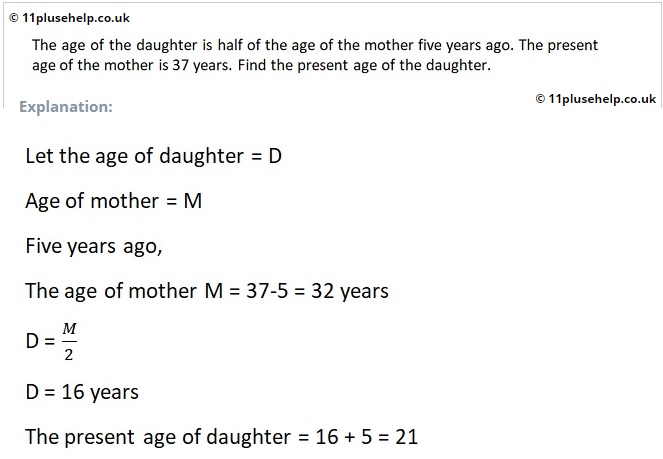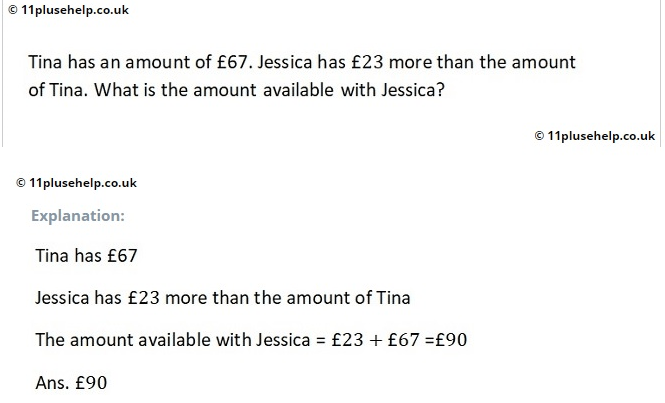# 11 Plus Maths Tests

Is your child facing difficulty solving 11 Plus School entrance Exams like GL Assessment, CEM, CSSE etc., conducted by different grammar schools, North London Schools, Independent Schools, and other private schools? Don’t worry, 11PluseHelp.co.uk helps your children overcome the maths test and top in the different school entrance exams. We provide online 11 plus test papers designed in three mediums: Easy, Medium, and Difficult to prepare every child from basics to advanced level.

11 Plus Maths Tests available @ 11PluseHelp.co.uk

We focus on fundamentals, logic, basics and cover most of the syllabus for all 11 Plus exam patterns in the UK. For example, We cover 11 Plus Maths papers topic wise questions and fundamentals and apply them. We have various test papers, time-based, short, long and various types, including standard mocks. All these tests lead to perfect practice, and the child should be fully prepared to face any 11 Plus Grammar School tests or Independent Schools tests.

We have detailed step by step explanations (self-explanatory) to all Maths questions, including many Independent schools past Maths papers. These tests are useful for the 11+ Exams preparation of CEM, CSSE, GL Assessment, Independent Schools, Grammar Schools and any other 11 Plus entrance examinations in the UK.

### 11 Plus Maths Topics:S.No 11 Plus Maths Tests 1 Coordinates 05 2 CSSE Maths 09 3 MixedMaths 02 4 Mixed Maths 01 5 Range 05 6 Coordinates 04 7 Measurement 05 8 Measurement 04 9 Profit and Loss 05 10 Measurement 03 11 Venn Diagrams 03 12 Maths Money 02 13 Profit and Loss 04 14 Maths Coordinates 03 15 Venn Diagrams 02 16 Measurement 02 17 Profit and Loss 02 18 Maths Range Test 03 19 Maths Inverse Operations 02 20 Measurements and Conversions 04 21 Maths Range Test 04 22 Negative Numbers 05 23 Negative Numbers 04 24 Maths Decimals 03 25 Maths Factors 04 26 Place Value and Rounding 05 27 Maths Factors 03 28 Maths Factors 02 29 Symmetry Test 04 30 Venn Diagrams 01 31 Triangle 03 32 Symmetry Test 03 33 Triangle 02 34 Maths Fractions 08 35 Maths Fractions 07 36 Nets of 3D Shapes Test 02 37 Profit and Loss 01 38 Data Tables 03 39 Data Tables 02 40 Maths Bodmas 05 41 Maths Bodmas 04 42 LCM HCF Test 03 43 Symmetry Test 02 44 Maths Mode 03 45 Maths Median 03 46 Place Value and Rounding 03 47 Place Value and Rounding 04 48 Function Machines Test 03 49 Maths Problem Solving 01 50 Pie charts and Bar charts 03 51 Units of Measurement 03 52 Arranging Numbers in Order 03 53 Negative Numbers 03 54 Ratio and Proportion Test 04 55 Arranging Numbers in Order 02 56 Measurements and Conversions 03 57 Maths Mode 02 58 Function Machines Test 02 59 Maths Time – Distance Test 02 60 Triangle 01 61 Units of Measurement 01 62 Units of Measurement 02 63 Measurements and Conversions 01 64 Measurements and Conversions 02 65 Pie charts and Bar charts 02 66 Maths Fractions 06 67 Maths Fractions 05 68 Pie charts and Bar charts 01 69 Data Tables 01 70 Maths Coordinates 01 71 Maths Angles Test 05 72 Maths Angles Test 04 73 Negative Numbers 02 74 Maths Mean Test 03 75 Percentages 03 76 Percentages 02 77 Maths Fractions 04 78 Short Maths Test 11 79 Place Value – Rounding test 02 80 Number Sequence Test 06 81 Maths 2D Shapes 02 82 Negative Numbers 01 83 Short Maths Test 10 84 Maths Fractions 03 85 Maths Decimals 02 86 Arranging Numbers in Order 01 87 Multiplication and Division 02 88 Multiplication and Division 01 89 Simple Math Calculations 07 90 Simple Math Calculations 06 91 Simple Math Calculations 05 92 Simple Math Calculations 04 93 Number Sequence Test 05 94 Simple Division Test 01 95 Simple MultiplicationTest 01 96 Simple Subtraction Test 01 97 Maths Demo Test 02 98 Simple Addition Test 01 99 Short Maths Test 09 100 Short Maths Test 08 101 Short Maths Test 07 102 Place Value – Rounding test 01 103 Number Sequence Test 04 104 Maths Inverse Operations 03 105 Simple Math Calculations 03 106 Function Machines Test 01 107 Nets of 3D Shapes Test 03 108 20 times Table 109 19 times Table 110 18 times Table 111 17 times Table 112 16 times Table 113 Simple Math Calculations 02 114 Simple Math Calculations 01 115 15 times Table 116 14 times Table 117 13 times Table 118 Maths Time – Distance Test 01 119 11 times Table 120 12 times Table 121 Maths Factors 01 122 10 times Table 123 9 times Table 124 8 times Table 125 7 times Table 126 6 times Table 127 5 times Table 128 4 times Table 129 3 times Table 130 2 times Table 131 Number Sequence Test 03 132 Maths Median 02 133 Number Sequence Test 02 134 Maths Area – Perimeter Test 03 135 Ratio and Proportion Test 03 136 Maths Angles Test 03 137 Maths Angles Test 02 138 Maths Angles Test 01 139 Maths Money and Cost Test 03 140 Maths Money and Cost Test 02 141 Maths 2D Shapes 01 142 Maths Area – Perimeter Test 02 143 Maths Inverse Operations 01 144 Ratio and Proportion Test 02 145 Ratio and Proportion Test 01 146 Nets of 3D Shapes Test 01 147 Symmetry Test 01 148 Number Sequence Test 01 149 Maths Area – Perimeter Test 01 150 LCM – HCF Test 02 151 LCM – HCF Test 01 152 Maths Money and Cost Test 01 153 Maths TimeCalculations Test 01 154 Percentages 01 155 Maths Fractions 02 156 Maths Decimals 01 157 Maths Median 01 158 Maths Range Test 02 159 Maths Range Test 01 160 Maths Mode 01 161 Maths Mean Test 02 162 Maths Algebra Test 06 163 Maths Algebra Test 03 164 Maths Mean Test 01 165 Short Maths Test 06 166 Bodmas Test 03 167 Maths Probability 02 168 Maths Algebra Test 02 169 Short Maths Test 05 170 Maths Bodmas 02 171 Maths Bodmas 01 172 Long Maths Test 05 173 Maths Money and Cost Test 04 174 Maths Fractions 01 175 Maths Area – Perimeter Test 04 176 Maths Algebra 01 177 Maths Probability 01 178 Long Maths Test 04 179 Short Maths Test 04 180 Long Maths Test 03 181 Long Maths Test 02 182 Maths Demo Test 01 183 Long Maths Test 01 184 Short Maths Test 03 185 Short Maths Test 02 186 Short Maths Test 01

11 Plus Maths Topic-Wise Free Practice Tests

Example problems from one of our 11 Plus Maths practice tests:

Example 1:Example2:Example3:11 Plus Mock Exam Papers

You can get information about all maths topics here:

https://www.11plusehelp.co.uk/11-plus-topics/11-plus-maths-topics

You can have unlimited online practice on 11 Plus subjects – Maths, English, Verbal Reasoning and Non-Verbal Reasoning.

11 Plus Topic-wise Free Practice Papers